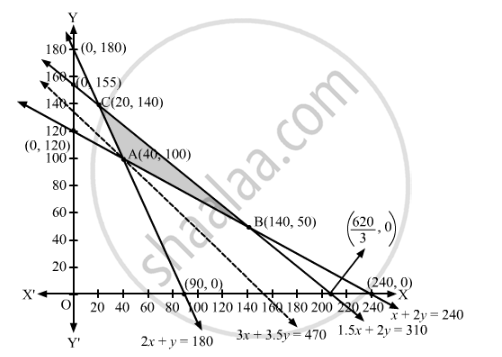# A Fruit Grower Can Use Two Types of Fertilizer in His Garden, Brand P and Q. the Amounts (In Kg) of Nirogen, Phosphoric Acid, Potash, and Chlorine in a Bag of Each Brand Are Given in the Table. - Mathematics

Sum

A fruit grower can use two types of fertilizer in his garden, brand P and Q. The amounts (in kg) of nirogen, phosphoric acid, potash, and chlorine in a bag of each brand are given in the table. Tests indicates that the garden needs at least 240 kg of phosphoric acid, at least 270 kg of potash and at most 310 kg of chlorine.

 kg per bag Brand P Brand P Nitrogen 3 3.5 Phosphoric acid 1 2 Potash 3 1.5 Chlorine 1.5 2

If the grower wants to minimize the amount of nitrogen added to the garden, how many bags of each brand should be used? What is the minimum amount of nitrogen added in the garden?

#### Solution

Let x bags of fertilizer of brand P and bags of fertilizer of brand Q are used in the garden.

One bag of brand P contains 3 kg of nitrogen and one bag of brand Q contains 3.5 kg of nitrogen, therefore, x bags of brand P and bags of brand Q will contain (3x + 3.5y) kg of nitrogen.

Since one bag of brand P contains 1 kg of phosphoric acid and one bag of brand Q contains 2 kg of phosphoric acid, therefore, x bags of brand P and bags of brand Q will contain (x + 2y) kg of phosphoric acid. But, the garden needs at least 240 kg of phosphoric acid.

∴ x + 2≥ 240

Similarly, x bags of brand P and bags of brand Q will contain (3x + 1.5y) kg of potash. But, the garden needs at least 270 kg of potash.

∴ 3x + 1.5y  ≥ 270

⇒ 2x + y  ≥ 180

Also, x bags of brand P and bags of brand Q will contain (1.5x + 2y) kg of chlorine. But, the garden needs at most 310 kg of chlorine.

∴ 1.5x + 2≤ 310

Thus, the given linear programming problem is

Minimise Z = 3x + 3.5y

subject to the constraints

x + 2≥ 240

2x + y  ≥ 180

1.5x + 2≤ 310

x, y ≥ 0

The feasible region determined by the given constraints can be diagrammatically represented as,The coordinates of the corner points of the feasible region are A(40, 100), B(140, 50) and C(20, 140).

The value of the objective function at these points are given in the following table.

 Corner Point Z = 3x + 3.5y (40, 100) 3 × 40 + 3.5 × 100 = 470   → Minimum (140, 50) 3 × 140 + 3.5 × 50 = 595 (20, 140) 3 × 20 + 3.5 × 140 = 550

The smallest value of Z is 470 which is obtained at (40, 100).

It can be seen that the open half-plane represented by 3x + 3.5y < 470 has no common points with the feasible region.

So, the minimum value of Z is 470.

Hence, 40 bags of fertilizer of brand P and 100 bags of fertilizer of brand Q are used in the garden. The minimum amount of nitrogen added in the garden is 470 kg.

Concept: Graphical Method of Solving Linear Programming Problems
Is there an error in this question or solution?

#### APPEARS IN

RD Sharma Class 12 Maths
Chapter 30 Linear programming
Exercise 30.3 | Q 15 | Page 40